Best Algebra and Trigonometry books to build a strong foundation in math field

Algebra and trigonometry present the essence of algebra and meets scope and sequence requirements for a typical introductory algebra and trigonometry course. By reading these books you will gain a solid understanding of the principles of trigonometry and how these concepts apply to real life.

Here you will get some books of Algebra and Trigonometry.

1. Algebra and Trigonometry Enhanced with Graphing Utilities (7th Edition)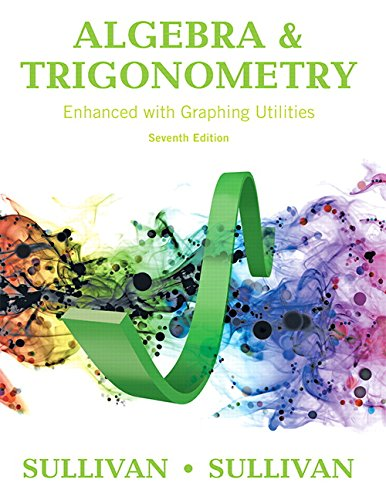Author: Michael Sullivan,Michael Sullivan III
Published at: 13/01/2016
ISBN: 0134119266

Algebra and Trigonometry Enhanced with Graphing Utilities is a very straightforward and easy to use math book. It includes real numbers, algebra essentials, geometry essentials, polynomials, factoring polynomials
synthetic division, rational expressions, nth roots; rational exponents in this guide. There is a chapter review
chapter test, cumulative review, chapter projects, prepare, practice, review at the end of every chapter of the book which will enable to figure out most of the exercises from the given examples and explanations.

Main coverage includes the following

• Graphs, equations, and inequalities
• Graphs
• Functions and their graphs
• Polynomial and rational functions
• Exponential and logarithmic functions
• Trigonometric functions
• Analytic Trigonometry
• Applications of trigonometric functions
• Polar coordinates; vectors
• Analytic geometry
• Systems of equations and inequalities
• Sequences; Induction; the binomial theorem
• Counting and probability.

2. Algebra and Trigonometry, Books A La Carte Edition Plus MyLab Math with eText -- Access Card Package (6th Edition)

The writer of this book Robert F. Blitzer is more direct in explaining things in a simpler term.

He provides step-by-step examples to help you pick up how things work. This is one of the best books for algebra and trigonometry that provides plenty of examples, which is something students tend to want above all else in a math book.

Here are some important coverage of this book

• Sequences, induction, and probability
• Conic sections and analytic geometry
• Matrices and determinants
• Systems of equations and inequalities
• Analytic Trigonometry
• Trigonometric functions
• Exponential and logarithmic functions
• Polynomial and rational functions
• Functions and graphs
• Equations and inequalities
• Prerequisites: fundamental concepts of algebra.

3. College Algebra and Trigonometry (6th Edition)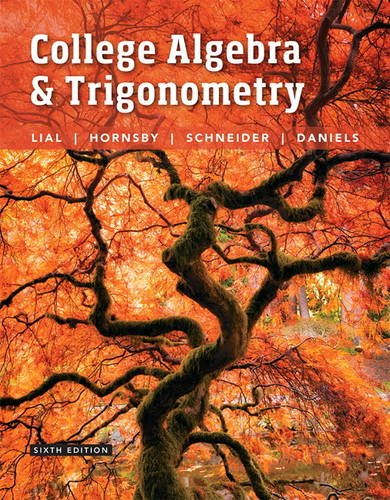Author: Margaret L. Lial,John Hornsby,David I. Schneider,Callie Daniels
Published at: 06/02/2016
ISBN: 0134112520

This guide reviews some basic concepts including sets, real numbers, and their properties, polynomials, factoring polynomials, rational expressions, rational exponents, radical expressions etc. This College Algebra and Trigonometry help students develop both the conceptual understanding and the analytical skills necessary for success in mathematics.

•  Equations and inequalities
• Graphs and functions
• Polynomial and rational functions
• Inverse, exponential, and logarithmic functions
• Trigonometric functions
• The Circular functions and their graphs
• Trigonometry further topics in algebra
• Identities and equations
• Applications of trigonometry
• Systems and matrices analytic geometry.

4. Algebra and Trigonometry: Graphs and Models (5th Edition)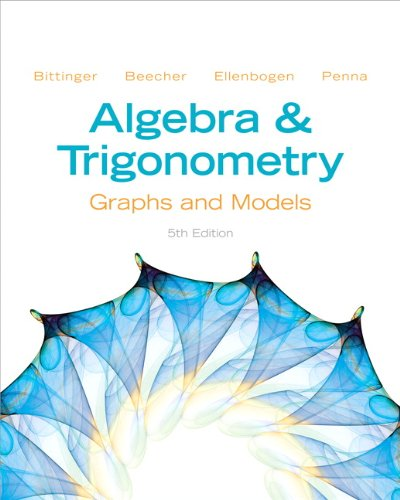Author: Marvin L. Bittinger,Judith A. Beecher,David J. Ellenbogen,Judith A. Penna
Published at: 29/01/2012
ISBN: 0321783972

Algebra and Trigonometry focus on functions, visual emphasis, side-by-side algebraic and graphical solutions, and real-data applications that will help students to get success.

It covers the following topics

• Rational expressions
• The basics of equation solving
• Factoring
• Addition, subtraction, and multiplication of polynomials
• Integer exponents, scientific notation, and order of operations
• The real-number system
• Radical notation and rational exponents.

5. Algebra and Trigonometry (5th Edition)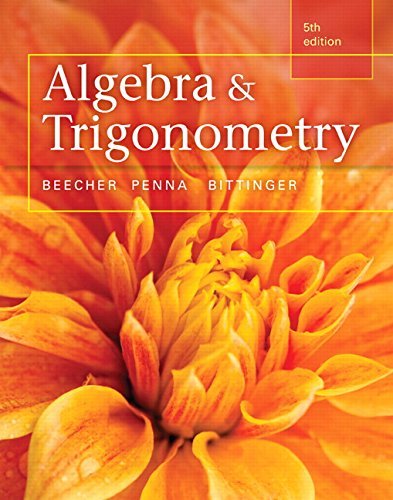Author: Judith A. Beecher,Judith A. Penna,Marvin L. Bittinger
Published at: 16/01/2015
ISBN: 0321969561

This is a great book with clear reading and examples. It helps the students how the math they are learning could apply to real life. This is the right book for you if you want to successfully do good in algebra and Trigonometry.

Important coverage are

• Graphs, functions, and models
• More on functions
• Inequalities
• Polynomial functions and rational functions
• Exponential functions and logarithmic functions
• The trigonometric functions
• Trigonometric identities, inverse functions, and equations
• Applications of Trigonometry
• Systems of equations and matrices
• Conic sections
• Sequences, series, and combinatorics.

6. College Algebra and Trigonometry, Books a la Carte Edition (3rd Edition)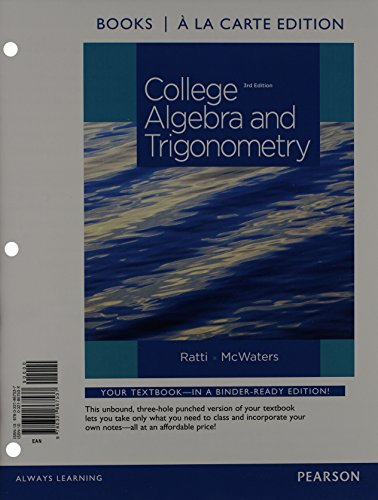Author: J. S. Ratti,Marcus S. McWaters
Published at: 25/01/2014
ISBN: 032186753X

This guide provides you the idea of some basic concepts of algebra including the real numbers and their properties, integer exponents and scientific notation, polynomials
factoring polynomials, rational expressions, and rational exponents and radicals. It will also help you by covering summary, review exercises, and practice test.

Contents include

• Equations and inequalities
• Graphs and functions
• Polynomial and rational functions
• Exponential and logarithmic functions
• Trigonometric functions
• Trigonometric identities and equations
• Applications of trigonometric functions
• Systems of equations and inequalities
• Matrices and determinants
• Conic sections
• Further topics in algebra.

7. Algebra and Trigonometry, Books a la Carte Edition (4th Edition)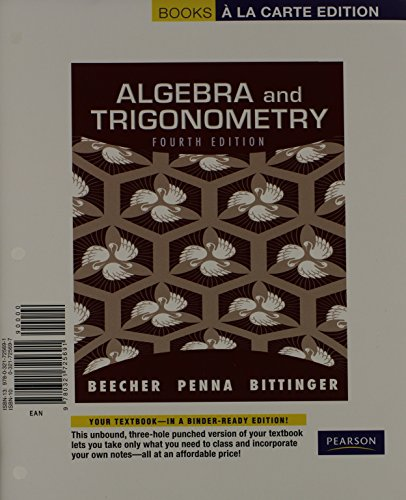Author: Judith A. Beecher,Judith A. Penna,Marvin L. Bittinger
Published at: 11/02/2011
ISBN: 0321725697

A very straightforward and clear book with plenty of practice problems and real-world examples. In this guide, the authors continue to innovate by incorporating more ongoing review to help students develop their understanding and study effectively.

This guide provides you

• Mixed review exercise sets
• Synthesizing concepts
• Built-in tools to help them prepare for tests.
• Vocabulary
• Example-based videos created by the authors
• Real world examples
• Pictures with large texts.

8. Algebra and Trigonometry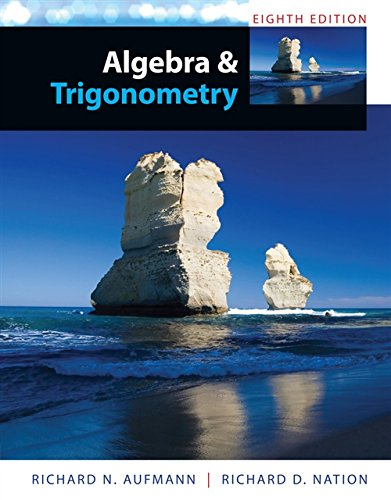Author: Richard N. Aufmann,Richard D. Nation
Published at: 01/01/2014
ISBN: 1285449428

A pleasant read that includes all of the important topics that are required in your class. This guide will help you to better your knowledge of algebra and trigonometry. This guide is highly recommended for students.

It provides

• Dynamic link between concepts and applications to bring mathematics to life
• Interactive learning techniques
• A better understanding of concepts and greater mathematical fluency.
• Technology features to accommodate courses that allow the option of using graphing calculators
• The key prerequisite skills needed to understand new concepts.

9. Trigonometry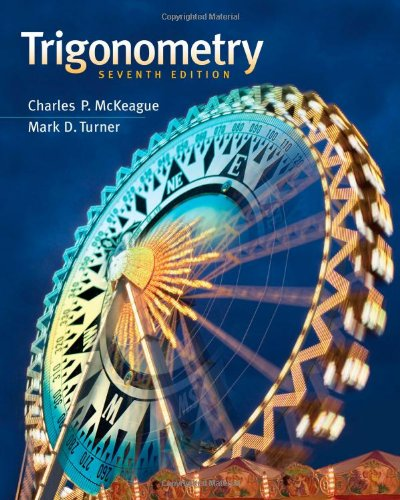Author: Charles P. McKeague,Mark D. Turner
Published at: 01/01/2012
ISBN: 1111826854

A very easy to follow guide with many exercises that explained thing in a logical order. If you are a self-learner this guide is must read for you. You will gain a solid understanding of the principles of trigonometry and how these concepts apply to real life. It also contains manageable sections using current, detailed examples and interesting applications.

Some of its coverage is

• The six Trigonometric functions
• Angles, degrees, and special triangles
• The rectangular coordinate system
• Trigonometric functions
• Introduction to identities
• More on identities
• Right triangle Trigonometry
• Calculators and Trigonometric functions of an acute angle
• Solving right triangles
• Applications
• Vectors: a geometric approach
• Reference angle
• Circular functions
• Arc length and area of a sector and much more.

10. Trigonometry

In this guide, Ron Larson provides 100 plus review questions for each section, answers to all odd-numbered questions, real-world applications, ongoing review, and innovative technology. There is a new Summarize features, Checkpoint problems, and a companion website reinforce understanding of the skill sets to help students better prepare for tests.

Important coverage are

• Review of real numbers and their properties
• Solving equations
• The Cartesian plane and graphs of equations
• Linear equations in two variables
• Functions
• Analyzing graphs of functions
• A library of parent functions
• Transformations of functions
• Combinations of functions
• Composite functions
• Inverse functions
• The unit circle
• Right triangle trigonometry
• Trigonometric functions of any angle and much more.

Thanks for reading this post. If you have any opinion don't hesitate to comment here. Also please subscribe our newsletter to get more updates.